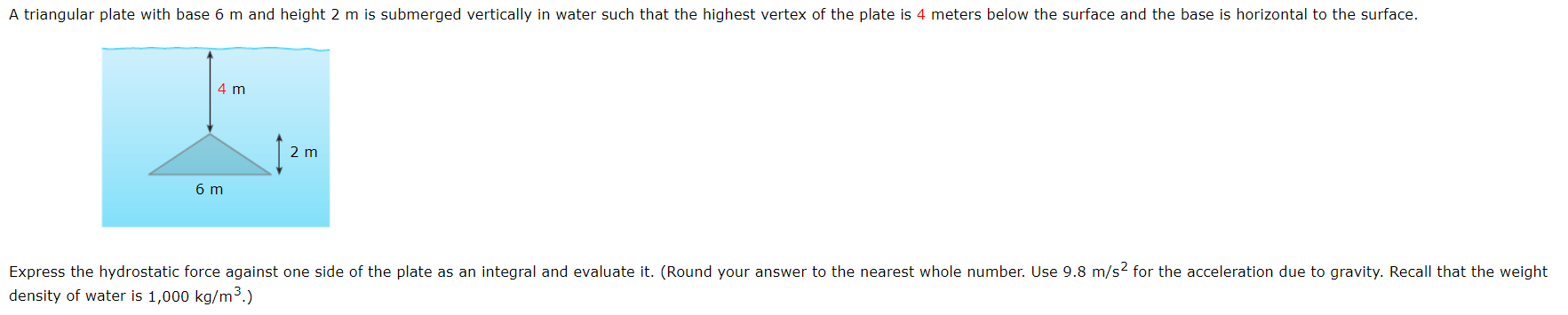# A triangular plate with base 6 m and height 2 m is submerged vertically in water such that the highest vertex of the plate is 4 meters below the surface and the base is horizontal to the surface4 m2 m6 mExpress the hydrostatic force against one side of the plate as an integral and evaluate it. (Round your answer to the nearest whole number. Use 9.8 m/s2 for the acceleration due to gravity. Recall that the weightdensity of water is 1,000 kg/m3.)

Question

Question in the attached image.help_outlineImage TranscriptioncloseA triangular plate with base 6 m and height 2 m is submerged vertically in water such that the highest vertex of the plate is 4 meters below the surface and the base is horizontal to the surface 4 m 2 m 6 m Express the hydrostatic force against one side of the plate as an integral and evaluate it. (Round your answer to the nearest whole number. Use 9.8 m/s2 for the acceleration due to gravity. Recall that the weight density of water is 1,000 kg/m3.) fullscreen
check_circleExpert Solution
Step 1

To determine the hydrostatic force against one side of the plate.

Step 2

Given:

Step 3

Concept Used:

The hydrostatic force on the one side of th...

### Want to see the full answer?

See Solution

#### Want to see this answer and more?

Solutions are written by subject experts who are available 24/7. Questions are typically answered within 1 hour*

See Solution
*Response times may vary by subject and question
Tagged in

### Calculus